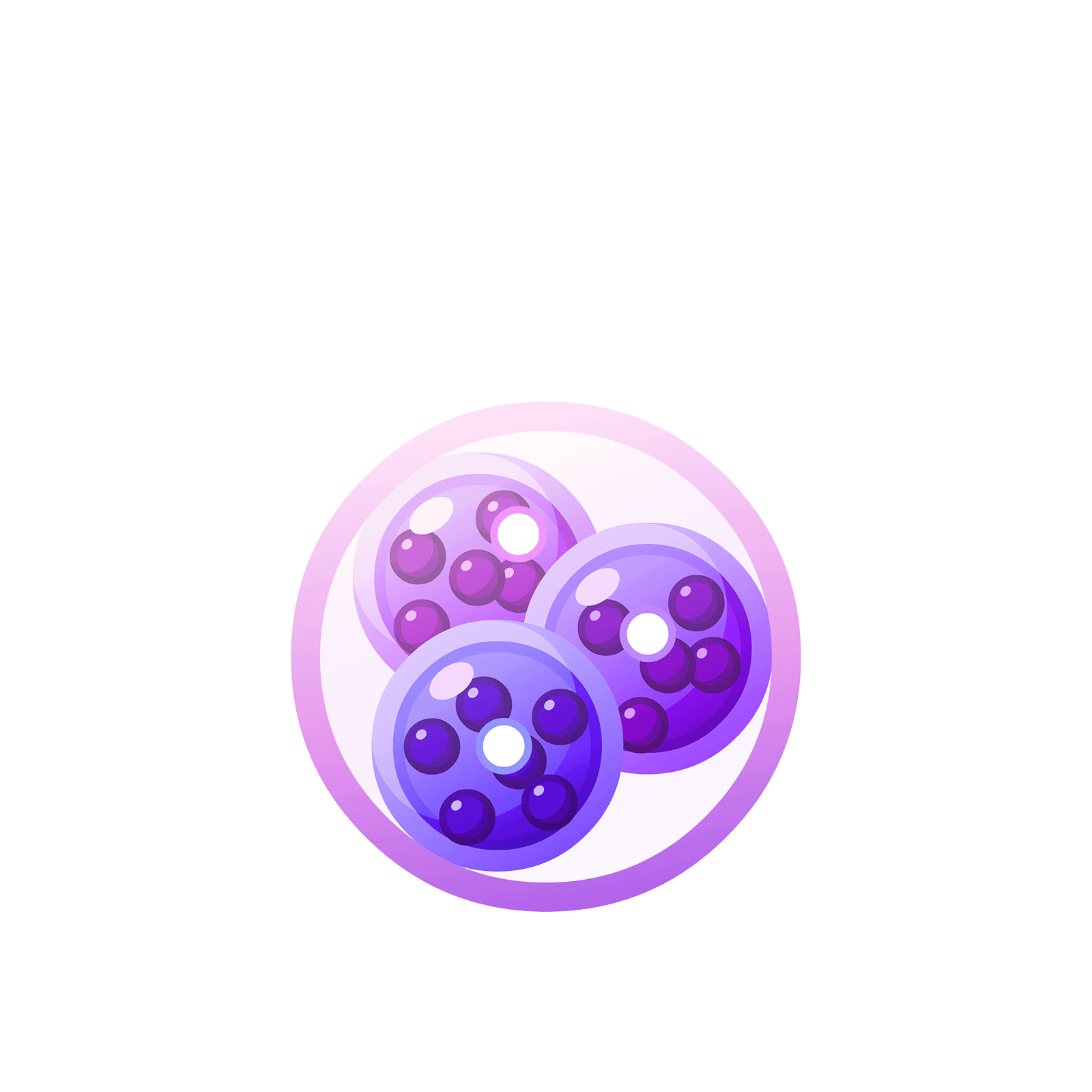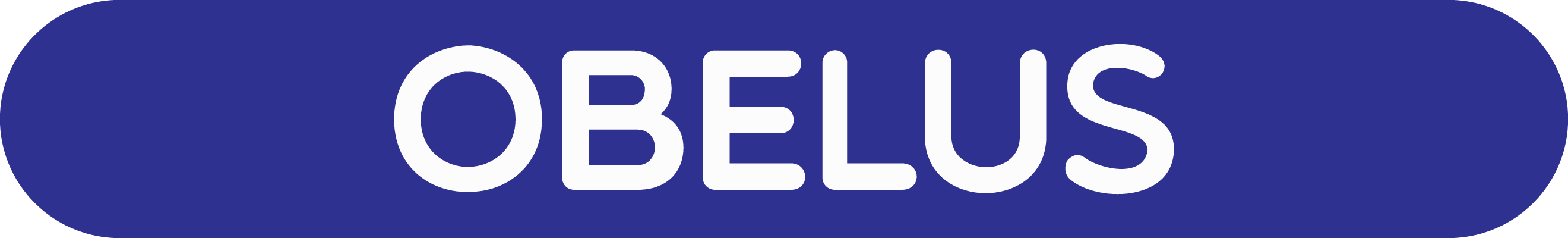05 217 385# Station  11DivisionHere’s an example of a division problem: Compute $276 \div 12$.

And here’s a horrible way to solve it: Draw a picture of $276$ dots on a page and then circle groups of twelve dots. You will see, after about an hour, that there are $23$ groups of twelve in a picture of $276$.

Here’s a great way to solve it: Draw a picture of $276$ dots in a $1 \leftarrow 10$ machine and just see right away that there are $23$ groups of twelve in it!

Read and play on to see how we can do this!

### Question 1

What is $3906\div 3$?

That is, how many groups of $3$ can we find in a picture of $3906$?

We can find $1$ at the thousands level, $3$ at the hundreds level, none at the tens level, and $2$ at the ones level.Try it!

Drag the group of three dots in the card at the bottom right onto the machine to find groups of three dots in the picture of $3906$.### Question 2

Calculate $402 \div 3$ using the $1 \leftarrow 10$ machine!### Question 3

Division by single-digit numbers is all well and good. What about division by multi-digit numbers? People usually call that long division.

Let’s consider the problem $276 \div 12$.

Here is the representation of $276$ in the $1 \leftarrow 10$ machine.We are looking for groups of twelve in this representation of $276$. Here’s what twelve looks like.Actually, this is not right as there would be an explosion in our $1\leftarrow 10$ machine. We need to always keep in mind that this really is a picture with all twelve dots residing in the rightmost box.Okay. So we’re looking for groups of $12$ in our picture of $276$. Do we see any one-dot-next-to-two-dots in the diagram?Yes. Here’s one.Can you find more groups of one-dot-next-to-two-dots in the machine?### Question 4

Try a few new cases to practice!

Use the dots-and-boxes approach to calculate $2783 \div 23$!### Question 5

A challenge:

Compute $3900 \div 12$.### Question 6

Let’s do another example. Let’s compute $31824 \div 102$.

Here’s the picture.Remember, all $102$ dots are physically sitting in the rightmost position of each set we identify.

Here’s my picture of the answer. I used different symbols for each group of $102$ that appears in $31824$. Does my picture make sense?There are $3$ groups | $1$ group | $2$ groups, that is, there are $312$ groups!

Try $31824 \div 102$ on the machine.### Question 7

Compute $46632 \div 201$.### Question 8

Here’s a tough challenge. It’s a problem that has a problem.
Can you make sense of the final answer you get?